# Santos-Lopez de Haro-Yuste hard disk equation of state

(diff) ← Older revision | Latest revision (diff) | Newer revision → (diff)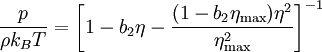$\frac{p}{\rho k_B T} = \left[ 1- b_2 \eta - \frac{(1-b_2 \eta_{\mathrm{max}}) \eta^2}{\eta^2_{\mathrm{max}}} \right]^{-1}$
where$p$ is the pressure,$\rho$ is the number density,$k_B$ is the Boltzmann constant,$T$ is the temperature,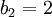$b_2=2$ is the reduced second virial coefficient,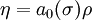$\eta = a_0(\sigma)\rho$ is the packing fraction, with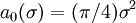$a_0(\sigma) = (\pi/4)\sigma^2$ the area of a hard disk with diameter$\sigma$, and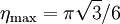$\eta_{\mathrm{max}} = \pi \sqrt3 /6$